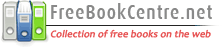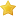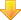Free Mathematics Books
 Abstract AlgebraLinear AlgebraCommutative AlgebraComplex AlgebraElliptic CurvesGeometric AlgebraGroups TheoryHigher AlgebraHomological AlgebraLie AlgebraDifferential AlgebraRings and FiledsGeometryAlgebraic GeometryDifferential GeometryFractalsRiemannian GeometryMathematical AnalysisComplex AnalysisFunctional AnalysisDifferential AnalysisFourier AnalysisHarmonic AnalysisNumerical AnalysisReal AnalysisTopologyAlgebraic TopologyDifferential TopologyGeometric TopologyK-theoryApplied MathematicsCalculusDifferential EquationsCombinatoricsDiscrete MathematicsGraph TheoryNumber TheoryProbability TheorySet TheoryTrigonometryCategory TheoryConstants & Numerical SequencesBasic MathematicsClassical AnalysisHistory of MathematicsArithmetic GeometryMathematical SeriesModern GeometryBasic AlgebraDifferential EquationsHome About Us Link to us Sitemap Contact Us Post QueriesOrdinary Differential Equations and Dynamical SystemsRatingsDownload / View bookOrdinary Differential Equations and Dynamical Systems
by

File Type :PDF
Number of Pages :364

Description
The aim of this book is to give a self contained introduction to the field of ordinary differential equations with emphasis on the dynamical systems point of view while still keeping an eye on classical tools as pointed out before. Covered topics are: Newton�s equations, Classification of differential equations, First order autonomous equations, Qualitative analysis of first order equations, Initial value problems, Linear equations, Differential equations in the complex domain, Boundary value problems, Dynamical systems, Planar dynamical systems, Higher dimensional dynamical systems, Local behavior near fixed points, Chaos, Discrete dynamical systems, Discrete dynamical systems in one dimension, Periodic solutions, Chaos in higher dimensional systems.Sponsored linksSimilar BooksArrayArrayArrayArrayArrayArrayArrayArrayArrayArrayArrayHome  |  About Us  |  Sitemap  |  Contact Us  |  Link to us  |  Post Your Queries |